Theory of one dimensional motion

For motion in one dimension, the distribution of the molecules over quantum states, speeds, and energies can be deduced.

Here we show that the energy of a macroscopic gas sample can be described on the basis of our knowledge of the quantum states allowed to the molecules of the gas and the distribution expressed by the Boltzmann expression. We begin by studying the translational motion in one dimension of a collection of molecules. You will see how the procedure is fascillated by the partition function.

Partition function: the molecules of a gas that move along one dimension can have, any of the energies given by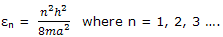For gas samples we can assume a cubic container and express a as V1/3, where V is the volume of the sample.

The partition function for one-dimensional translational motion can be developed by recognizing that

The translational energy of the lowest-energy state is small compared with the energies of most of the populated states and can be set equal to zero.

The translational-energy spacing between successive energy levels is small compared with the range of energies of the populated states.

The degeneracy of each energy level is unity.

On this basis, the partition function summation over the translational energies can be replaced by integration, and the partition function is expressed asThe integral is one of the definite integrals dealt by using the general result shown there, we obtain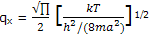Example: calculate the partition function for the translational motion of N2 molecules free to move along one dimension of a 1-L cubic container. The temperature is 25°C.

Solution: the translational-energy factor h2/(8ma2) can be calculated conveniently from the expression of this equation. The mass of M of 1 mol of N2 molecules is 0.02801 kg, and V = 1 L = 10-3 m3. Thus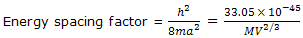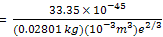= 1.180 × 10-40 J

The value of kT, to which the energy spacing factor is compared, is

kT = (1.3807 × 10-23 J K-1) (298.15 K) = 4.116 × 10-21 J

The partition function is calculated as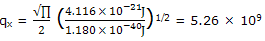this large partition function value indicates that very many states are available to the molecules. This result, in the calculations, from the smallness of h2/(8ma2compared to kT.

Average energy: the one dimensional translational energy of 1 mol of gas molecules can now be deduced. The general thermal-energy expression is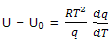The partition function for one-dimensional translational motion gives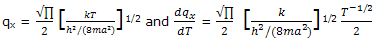substitution of the equation expressions in the equation for U - U0 gives

U - U0 = ½ RT

We have come by this long route to the result that we obtained from the simple classical kinetic-molecular theory. The translational energy per degree of freedom is ½ RT

#### Related Questions in Chemistry

• ##### Q :Problem on vapour pressure Choose the

Choose the right answer from following. If P and P are the vapour pressure of a solvent and its solution respectively N1 and N2 and are the mole fractions of the solvent and solute respectively, then correct relation is: (a) P= PoN1 (b) P= Po N2 (c)P0= N2 (d)

• ##### Q :Homework Silicon has three naturally

Silicon has three naturally occurring isotopes. 28Si, mass = 27.976927; 29Si, mass = 28.976495; 30Si, mass = 29.973770 and 3.10% abundance. What is the abundance of 28Si?

• ##### Q :Question related to colligative

The colligative properties of a solution depend on: (a) Nature of solute particles present in it (b) Nature of solvent used (c) Number of solute particles present in it (d) Number of moles of solvent only

• ##### Q :Molecular Diameters The excluded volume

The excluded volume b, introduced by vander Wall's as an empirical correction term, can be related to the size gas molecules. To do so, we assume the excluded volume is the result of the pairwise coming together of molecules. This assumption is justified when b values

• ##### Q :Colligative property associated question

Give me answer of this question. Which of the following is not a colligative property : (a)Optical activity (b)Elevation in boiling point (c)Osmotic pressure (d)Lowering of vapour pressure

• ##### Q :Eutectic Formation In some two

In some two component, solid liquid systems, a eutectic mixture forms.Consider, now a two component system at some fixed pressure, where the temperature range treated is such as to include formation of one or more solid phases. A simple behavior is shown b

• ##### Q :Which solution will have highest

Which solution will have highest boiling point:(a) 1% solution of glucose in water  (b) 1% solution of sodium chloride in water  (c) 1% solution of zinc sulphate in water  (d) 1% solution of urea in waterAnswer: (b) Na

• ##### Q :Vapour pressure over mercury Choose the

Choose the right answer from following. At 300 K, when a solute is added to a solvent its vapour pressure over the mercury reduces from 50 mm to 45 mm. The value of mole fraction of solute will be: (a)0.005 (b)0.010 (c)0.100 (d)0.900

• ##### Q :Describe various systems for

Common system According to this system, the individual members are named according to alkyl groups att

• ##### Q :Lowering of vapour pressure Help me to

Help me to go through this problem. Lowering of vapour pressure is highest for: (a) urea (b) 0.1 M glucose (c) 0.1M MgSo4 (d) 0.1M BaCl2